## Part I

Repeat the Examples 1-8

## Part II

1. State the logarithms of these numbers, to three decimal places:
1. 452.6
2. 0.2
3. 1,000
4. 4.5×1015
5. 0.00724
6. 6.4×10-8
7. 7.66
2. State the logarithms of these numbers, to three decimal places:
1. 15,250
2. 0.0001
3. 1.4×10-5
4. 1.11
5. 200
6. 7.5×1012
7. 0.000215
3. What values have the following logarithms (round answers to three significant digits):
1. 3.526
2. 0.01
3. -2.769
4. 10
5. -0.168
6. 5.728
7. 0
4. What values have the following logarithms (round answers to three significant digits):
1. -2
2. 1.592
3. 5.923
4. -4.511
5. -1.735
6. 0.301
7. 23.301
5. Which of these values has a logarithm greater than 1?
1. 45.8
2. 8.3
3. 195,680
4. -15.2
5. 0.783
6. 5.4×1012
7. 10
6. Which of these values has a logarithm less than 1?
1. 6.8
2. 55.5
3. 4.2×109
4. 0.00002
5. 75
6. 9.27×10-3
7. 10
7. Which of the graphs below indicate that the corresponding dataset follows an exponential pattern?
 Graph A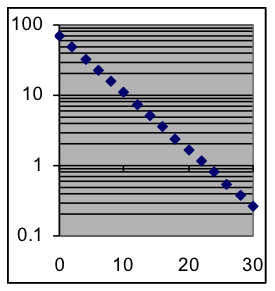Graph B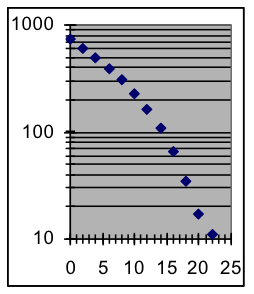Graph C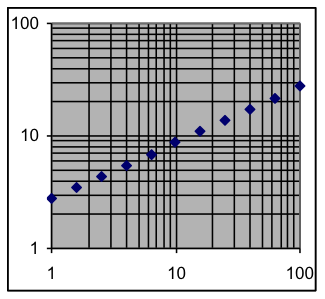8. Which of the graphs above indicate that the corresponding dataset follows a power-function pattern?
9. Use a semi-log graph to show whether dataset A is exponential.The point halfway between the x=1 and x=2 data points has a y value that is about halfway between y=100 and y=200.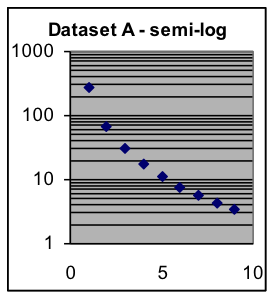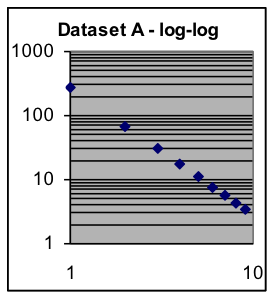Dataset A x Y 1 275.3 2 68.8 3 30.6 4 17.2 5 11.0 6 7.6 7 5.6 8 4.3 9 3.4
 Dataset B x y 20 4 40 16 60 36 80 64 100 100 120 144 140 196 160 256 180 324

10. Use a semi-log graph to show whether dataset B is exponential.
11. Use the Exercise 17 graph to interpolate an estimated value for x = 1.5.
12. Use the Exercise 18 graph to interpolate an estimated value for x = 170.
13. Use a log-log graph to tell if dataset A approximates a power function.
14. Use a log-log graph to tell if dataset B approximates a power function.
15. Scientists have found that the total energy requirements of animals increase somewhat more slowly than body size. For example, a 1.2-pound mongoose requires 47 kilocalories per day, a 10-pound fox requires 240, a 22-pound bobcat requires 440, a 100-pound wolf requires 1350, a 300-pound lion requires 3100, a 400-pound tiger requires 3800, and a 700-pound polar bear requires 5900.
1. What model is appropriate for predicting energy requirement from weight? (You will need to decide on the type of model, then find the best-fit parameters to this data for that type.)
2. What daily energy requirement can be expected for a 45-pound lynx?
Infant data averages for Exercises 24–28
Age
(months)
Weight
(pounds)

Length

(inches)

3 13.0 24.0
6 17.2 26.7
9 20.3 28.6
12 22.2 30.0
15 24.0 31.4
18 25.3 32.5
21 26.6 33.6
24 27.8 34.5
(US Natl Cen Health Stat)
1. Fit a power-function model to the infant data shown above, using age as the input variable and average weight as the output variable.
1. State the best-fit scale and power parameters in an appropriate model formula.
2. Is this model a good fit to the data?
3. Compute the predicted average infant weight for an age of 20 months.
2. Fit a power-function model to the infant data shown above, using average weight as the input variable and age as the output variable.
1. State the best-fit scale and power parameters in an appropriate model formula.
2. What is the relationship of the power parameter in this model with the power parameter of the model found in the previous problem? Why?
3. Fit a power-function model to the infant data shown above, using age as the input variable and average length as the output variable.
1. State the best-fit scale and power parameters in an appropriate model formula.
2. Is this model a good fit to the data?
3. Compute the predicted average infant length for an age of 20 months.
4. Fit a power-function model to the infant data shown above, using average length as the input variable and average weight as the output variable.
1. State the best-fit scale and power parameters in an appropriate model formula.
2. Use the predicted average infant length for an age of 20 months (computed in section c of the previous exercise) as input to the model found in this exercise, producing as output a predicted infant weight for an age of 20 months. What earlier exercise also predicted this quantity? How well do the predictions match?
5. Using the results of the previous exercise (without doing any more fitting), use a single computation to estimate what the best-fit power parameter would be for a model based on this data that predicts average length from average weight.